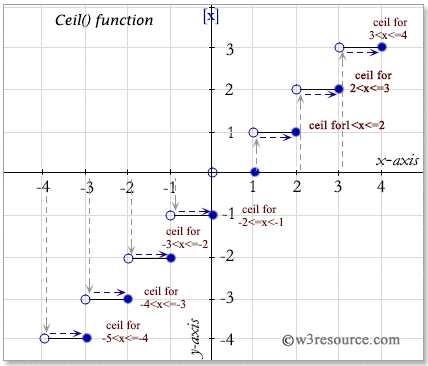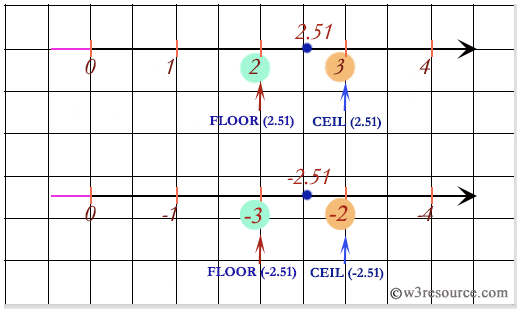# SQL CEIL() function

## CEIL() function

SQL CEIL() function is used to get the smallest integer which is greater than, or equal to, the specified numeric expression.

Syntax:

`CEIL(expression)`

Parameters:

Name Description
expression An expression which is a numeric value or numeric data type.The bit data type is not allowed.

MySQL, PostgreSQL, and Oracle

All of above platforms support the SQL syntax of CEIL().

## SQL CEIL() function: Pictorial presentation## SQL CEIL() function on positive value

To get the ceiling or nearest rounded up value of 17.36 from the DUAL table , the following SQL statement can be used :

```SELECT(CEIL(17.36))
FROM dual;
```

Output:

```(CEIL(17.36))
-------------
18
```

Example:

To get the ceiling or nearest rounded up value of -17.36 from the DUAL table, the following SQL statement can be used :

``````SELECT CEIL(-17.36)
FROM dual;
``````

Output:

```CEIL(-17.36)
------------
-17
```

## SQL CEIL() function with distinct

Sample table : agents

To get the unique ceiling or nearest rounded up value of the column 'commission' after multiplying by 75 from the 'agents' table, the following SQL statement can be used :

``````SELECT DISTINCT(CEIL(commission*75)) "DISTINCT(CEIL())"
FROM agents;
``````

Output:

```DISTINCT(CEIL())
----------------
11
10
9
12
```

## SQL: Comparing between CEIL() and FLOOR() functionNote: Outputs of the said SQL statement shown here is taken by using Oracle Database 10g Express Edition.

Here is a slide presentation which covers the SQL arithmetic functions.

Practice SQL Exercises